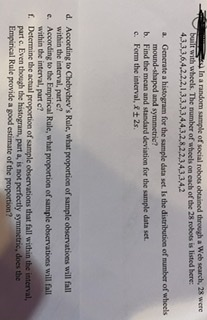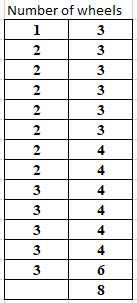# In a random sample of social robots obtained through a Web search, 28 werebuilt with wheels. The number of wheels on cach of the 28 robots is listed here:4,3,33,6,4,2,2,2,1,3,3,3,3,4,4,3,2,8,2,2,3,43,3,4,2a Generate a histogram for the sample data set. Is the distribution of number of wheelsmound-shaped and symmetrie?b. Find the mean and standard deviation for the sample data set.c. Form the interval, &t 2s.d. According to Chebychev's Rule, what proportion of sample observations will fallwithin the interval, part e?e. According to the Empirical Rule, what proportion of sample observations will fallwithin the interval, part c?f. Determine the actual proportion of sample observations that fall within the interval,part e. Even though the histogram, part a, is not perfectly symmetric, does theEmpirical Rule provide a good estimate of the proportion?

Question
22 views

Part D&E&Fhelp_outlineImage TranscriptioncloseIn a random sample of social robots obtained through a Web search, 28 were built with wheels. The number of wheels on cach of the 28 robots is listed here: 4,3,33,6,4,2,2,2,1,3,3,3,3,4,4,3,2,8,2,2,3,43,3,4,2 a Generate a histogram for the sample data set. Is the distribution of number of wheels mound-shaped and symmetrie? b. Find the mean and standard deviation for the sample data set. c. Form the interval, &t 2s. d. According to Chebychev's Rule, what proportion of sample observations will fall within the interval, part e? e. According to the Empirical Rule, what proportion of sample observations will fall within the interval, part c? f. Determine the actual proportion of sample observations that fall within the interval, part e. Even though the histogram, part a, is not perfectly symmetric, does the Empirical Rule provide a good estimate of the proportion? fullscreen
check_circle

Step 1

(Here even though 28 ROBOTS mentioned in question, there are only 27 data points. So considering there are only 27 robots* and performing the calculations.

d)
Given data set of number of wheels each of 27 robots haveAccording to Chebyshev's rule
for any k > 1, at least (1-1/k2 )of the data lies within k standard deviations of the mean.
Here the interval in part c is given with in two standard deviations. So k = 2 we get (1 - 1/4) = 0.75.
So we say at least 75% of the data lie with in two standard deviations of the mean.

Step 2

e)
According to Empirical rule
With in one,two and three standard deviations of the mean 68%,95% and 99.7% of the data lie respectively.

The g...

### Want to see the full answer?

See Solution

#### Want to see this answer and more?

Solutions are written by subject experts who are available 24/7. Questions are typically answered within 1 hour.*

See Solution
*Response times may vary by subject and question.
Tagged in

### Statistics